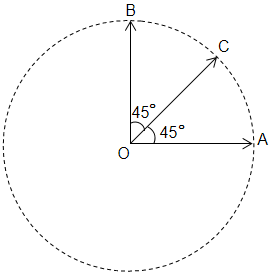NEET Physics Mathematical Tools Questions Solved

The three vectors OA, OB and OC have the same magnitude R. Then the sum of these vectors have magnitude -(A)  $\mathrm{R}$

(B)  $\sqrt{2}\mathrm{R}$

(C)  $3\mathrm{R}$

(D)  $\left(1+\sqrt{2}\right)\mathrm{R}$

Concept Videos :-

#20 | Vectors: Introduction
#21 | Vectors: Addition
#22 | Vectors : Subtraction

Concept Questions :-

Resultant of Vectors
Explanation is a part of a Paid Course. To view Explanation Please buy the course.

Difficulty Level: